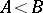# Archimedean axiom

(diff) ← Older revision | Latest revision (diff) | Newer revision → (diff)
An axiom, originally formulated for segments, which states that if the smaller one of two given segments is marked off a sufficient number of times, it will always produce a segment larger than the larger one of the original two segments. This axiom can be formulated in an analogous manner for surfaces, volumes, positive numbers, etc. In general, the Archimedean axiom applies to a given quantity if for any two valuesandof this quantity such thatit is always possible to find an integersuch that. The axiom forms the base of the process of successive division in arithmetic and in geometry (cf. Euclidean algorithm). The importance of the Archimedean axiom only became fully apparent after the discovery, in the 19th century, of magnitudes to which it does not apply — so-called non-Archimedean quantities (cf. Quantity; see also Archimedean group; Archimedean ring; Archimedean class).